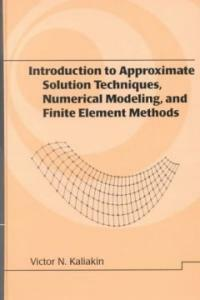> 상세정보

상세정보Introduction to approximate solutions techniques, numerical modeling, and finite element methods (3회 대출)

자료유형
단행본
개인저자
Kaliakin, Victor N.
서명 / 저자사항
Introduction to approximate solutions techniques, numerical modeling, and finite element methods / Victor N. Kaliakin.
발행사항
New York :   Marcel Dekker,   2002.
형태사항
xvi, 674 p. : ill. ; 24 cm.
총서사항
Civil and environmental engineering ; 9
ISBN
082470679X (alk. paper)
서지주기
Includes bibliographical references (p. 613-63) and index.
일반주제명
Finite element method.
 000 00881camuu22002534a 4500 001 000000769301 005 20020611103525 008 010724s2002 nyua b 001 0 eng 010 ▼a 01047122 020 ▼a 082470679X (alk. paper) 040 ▼a DLC ▼c DLC ▼d 211009 042 ▼a pcc 049 1 ▼l 121063406 ▼f 과학 050 0 0 ▼a TA347.F5 ▼b K35 2002 082 0 0 ▼a 620/.001/51535 ▼2 21 090 ▼a 620.0015 ▼b K14i 100 1 ▼a Kaliakin, Victor N. 245 1 0 ▼a Introduction to approximate solutions techniques, numerical modeling, and finite element methods / ▼c Victor N. Kaliakin. 260 ▼a New York : ▼b Marcel Dekker, ▼c 2002. 300 ▼a xvi, 674 p. : ▼b ill. ; ▼c 24 cm. 440 0 ▼a Civil and environmental engineering ; ▼v 9 504 ▼a Includes bibliographical references (p. 613-63) and index. 650 0 ▼a Finite element method.

소장정보

No. 소장처 청구기호 등록번호 도서상태 반납예정일 예약 서비스
No. 1 소장처 청구기호 620.0015 K14i 등록번호 121063406 도서상태 대출가능 반납예정일 예약 서비스

컨텐츠정보

목차

Governing equations and their approximate solution; computer storage and manipulation of numbers; the finite difference method; the method of weighted residuals; variational methods; introduction to the finite element method; development of finite element equations; steps in performing finite element analysis; element interpolation functions; element mapping; finite element analysis of scalar field problems; finite element analysis in linear elastostatics; implementation, modelling, and related issues. Appendices: mathematical potpourri; matrices and linear algebra; some notes on heat flow; local and natural coordinate systems; the patch test; solution of linear systems of equations; notes on integration of finite elements.

관련분야 신착자료

솔리드이엔지 (2021)

(Python을 이용한) 공학수치해석 / 수정판

Kiusalaas, Jaan (2021)

이상만 (2021)

김정원 (2021)

마스터링 리버스 엔지니어링 : 소프트웨어 취약점을 분석하는 해커 친화적 기술

Wong, Reginald (2021)

Nanopore technology : methods and protocols

Fahie, Monifa A. V (2021)

김흥수 (2021)

기계재료 편람 : 금속 및 비금속재료 KS규격 핸드북

테크노공학기술연구소 (2021)